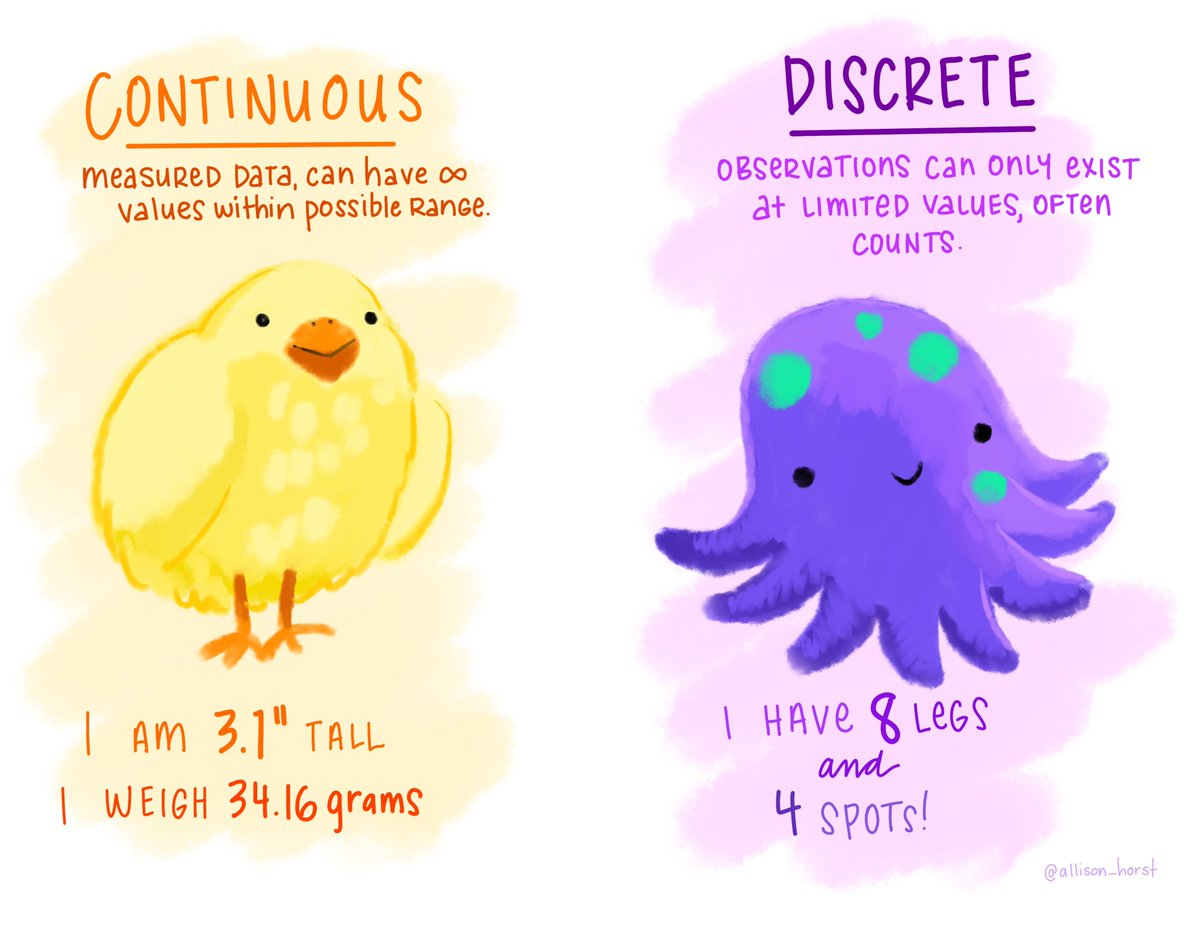September 22 2022

## Agenda

• Variable types
• Mapping variable to plot elements
• Bar plots with Vega

## Numeric Variables

Numeric variables are also known as quantitative variables.Illustration by Alisson Horst (https://github.com/allisonhorst/stats-illustrations)

## Numeric Variables

Can you think of examples of these two types of numeric variables?

• Continuous numeric variables
• Discrete numeric variables

What are good visualization mappings (or encodings) for continuous and discrete numeric variables?

## Categorical Variables

Categorical variables are also known as qualitative or nominal variables.